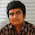## Welcome to the World of Modelling and Simulation

### What is Modelling?

This blog is all about system dynamics modelling and simulation applied in the engineering field, especially mechanical, electrical, and ...### Explicit Euler Method to Solve System of ODEs in MATLAB

In this tutorial, I am going to show a simple way to solve system of first order ordinary differential equations (ODE) by using explicit Euler method. Let' say, we have following three first order ODEs.

Here, we have 3 ODEs, 3 dependent variables (x, y, z), and 1 independent variable, t. The initial conditions when time is zero are, x(0) = y(0) = z(0) =1. Our goal is to solve these differential equations with explicit Euler approach and plot the solutions afterwards.

Step 1: Define the Equations
The first step is to define all the differential equations in MATLAB. I did this by using MATLAB function handle, which is shown below.

Step 2: Choose a Numerical Approach
The next step is to select a numerical method to solve the differential equations. In this example, we will use explicit Euler method. I have created a function to implement the algorithm. The following image shows the application of the explicit Euler method.

Step 3: Call the Function
This is the final step where we need to call the function. In the previous step, we have created a function, which we are going to call in this step to solve the equations.

So, that's it. We are done with the process, and we can now visualize the solution. I have also attached the MATLAB codes for this problem at the end.

MATLAB Program:

close all;

clc;
format long;

% Defining the three differential equations of the problem
f = @(t,y) [-5*y(1)+5*y(2); 14*y(1)-2*y(2)-y(1)*y(3); -3*y(3)+y(1)*y(2)];
[x,y] = explicit_euler(f,[0,5],[1;1;1],0.001); % Calling Euler function
plot(x,y);
title('When Time Step is 0.001');
legend('x(t)', 'y(t)', 'z(t)', 'Location', 'NorthEast')
xlabel('t')
ylabel('Solutions')

function [x, y] = explicit_euler( f, xRange, y_initial, h )
% This function uses Euler’s explicit method to solve the ODE
% dv/dt=f(t,v); x refers to independent and y refers to dependent variables
% f defines the differential equation of the problem
% xRange = [x1, x2] where the solution is sought on
% y_initial = column vector of initial values for y at x1
% numSteps = number of equally-sized steps to take from x1 to x2
% x = row vector of values of x
% y = matrix whose k-th column is the approximate solution at x(k)
x(1) = xRange(1);
numSteps = ( xRange(2) - xRange(1) ) /h ;
y(:,1) = y_initial(:);
for k = 1 : numSteps
x(k + 1) = x(k) + h;
y(:,k+1) = y(:,k) + h * f( x(k), y(:,k) );
end

#ExplicitEulerMethod #Matlab #NumericalMethod #Blog #Blogger

1.I have system of 12 coupled ode's with discrete data imported from excel. how to interpolate this data and integrate my system using Euler's method?
discrete data : T,m,rxA,Ixx,Iyy,Izz (vary with time)

1.Hi, you can follow the Euler's method implementation by Matlab from this blog post. At first, you need to write your 12 coupled ODEs. Make sure that are in first order form, if not convert them. Next, define your variables. You can import the data in Matlab from your excel sheet. Finally, call the Euler's method function (for example, shown in this tutorial) to solve the coupled equations. Let me know if you have any questions?

2.Awesome. This tutorial has helped me much. Thanks indeed

3.I am very glad to know Vatige, thanks for your feedback :)

4.The code is not working.
function [x, y] = explicit_euler( f, xRange, y_initial, h )
Error is at y_initial
Error: File: ODEsolution.m Line: 16 Column: 1
Function definitions are not permitted in this context.